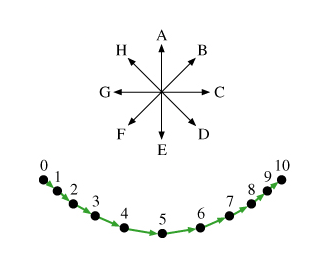# Problem: The motion diagram shown in the figure represents a pendulum released from rest at an angle of 45° from the vertical. The dots in the motion diagram represent the positions of the pendulum bob at eleven moments separated by equal time intervals. The green arrows represent the average velocity between adjacent dots. Also given is a "compass rose" in which directions are labeled with the letters of the alphabet.A. What is the direction of the acceleration of the object at moment 5? (Enter the letter of the arrow with this direction from the compass rose in the figure. Type Z if the acceleration vector has zero length.)B. What is the direction of the acceleration of the object at moments 0 and 10? (Enter the letters corresponding to the arrows with these directions from the compass rose in the figure, separated by commas. Type Z if the acceleration vector has zero length.)

###### FREE Expert Solution

A.

The force of gravity at point 5 is acting downwards, while the tension is pulling the bob upwards.

Therefore, there is no tangential force acting on the bob.

84% (53 ratings)###### Problem Details

The motion diagram shown in the figure represents a pendulum released from rest at an angle of 45° from the vertical. The dots in the motion diagram represent the positions of the pendulum bob at eleven moments separated by equal time intervals. The green arrows represent the average velocity between adjacent dots. Also given is a "compass rose" in which directions are labeled with the letters of the alphabet.A. What is the direction of the acceleration of the object at moment 5? (Enter the letter of the arrow with this direction from the compass rose in the figure. Type Z if the acceleration vector has zero length.)

B. What is the direction of the acceleration of the object at moments 0 and 10? (Enter the letters corresponding to the arrows with these directions from the compass rose in the figure, separated by commas. Type Z if the acceleration vector has zero length.)